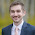This page demonstrates Python tips and tricks that I use in my everyday programming as an atmospheric science graduate student.
-Brian Blaylock

## Tuesday, August 2, 2016

### 🐍 An updated notebook is available for this demonstration on GitHub.

Also look at my demonstration using the KDTree method ( to find nearest neighbor for many points.

I deal a lot with weather model output (specifically HRRR model data) and often need to verify a data point with observational data. Weather stations we get observations from are not located at the exact grid points as the model's grid points. The hard part is finding the index value of a latitude and longitude grid point nearest to the observation location. This is made easy with numpy's maximum function (not max).

First, you need to have three arrays, one for latitude, one for longitude, and one for the environment variable such as temperature. Load these values into a numpy array. I use pygrib to read model output in grib2 format.

lat = latitude  # a 2D numpy array of your latitudes
lon = longitude # a 2D numpy array of your longitudes
temp = temperature # a 2D numpy array of your temperatures, or other variable

Next you need to know the latitude and longitude for the observation point. We are looking for the nearest grid point in the lat and lon arrays for that grid point.

stn_lat = station's latitude # eg. 40
stn_lon = station's longitude # eg. -110

Now find the absolute value of the difference between the  station's lat/lon with every point in the grid. This tells us how close a point is to the particular latitude and longitude.

abslat = np.abs(lat-stn_lat)
abslon= np.abs(lon-stn_lon)

If you plot abslat and abslon, you'll see what we have done...we have created two new arrays with that tell us how close each latitude and longitude is to the grid point we are interested in.abslat: the blue line represents the nearest grid latitude line our station is located.abslon: the blue line represents the nearest grid longitude our station is located.
Now we need to combine these two results.
We will use numpy.maximum, which takes two arrays and finds the local maximum.

c = np.maximum(abslon,abslat)

Which is an array that looks like this...c: an array expressing the local maximums between the abslat and abslon arrays
The dark blue point is the nearest grid location to our station. You can find the index location on the grid of this by using the min function.

latlon_idx = np.argmin(c)

Now, this latitude/longitude index value is the index for a flattened array, so when you look for that same index value in, say, your temperature array, you should flatten the array to pluck out the value at that point.

grid_temp = temp.flat[latlon_idx]

If you don't like flattened arrays, you can also get the row/column index like this
x, y = np.where(c == np.min(c))
grid_temp = temp[x, y

And there you have it!

1.Hi, Brain its a nice example.
I also have the similar problem that I have the netcdf file in which it contains the longitude, latitude and temperature data and I would like to verify with the cyclone track positions with the netcdf file locations (track positions are not located at the exact grid points as the netcdf data) and extract the following temperature data from the netcdf data. I followed your procedure, but the problem is that my netcdf file does not contain the equal longitude and latitude values.
Could you please help me how to sort out this issue and if you want I can send my code to you for your reference.

2.Nice post Brian!
I did not understand why you used c = np.maximum(abslon,abslat) and then latlon_idx = np.argmin(c). Would you please elaborate?

3.np.maximum will find the maximum value between the two grids, abslon and abslat. The minimum value of the grid I labeled "c" is the point nearest the point of interest. To identify the location in the grid where the minimum was, I used np.argmin.

4.Hi,this is super useful!
I would also like to have the 2nd, 3rd and 4th closest grid points...
Is that possible with this method ?

5.Where are you getting a definitive list of all the weather station latlons?

6.@Litmon, You can get public weather station data from the Synoptic API: https://developers.synopticdata.com/mesonet/